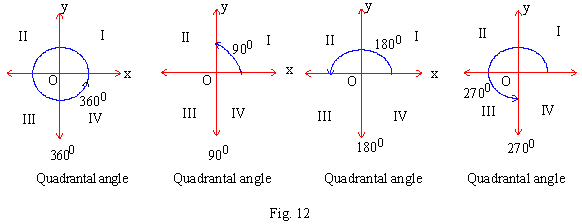Introduction:
In order to become a more advance math student, it is necessary to understand the basics behind the trigonometry of angles. There are even some cases where there are shortcuts to make your life easier, but more on that later.
Reference Angles:
Reference angles allow us to evaluate more complex angles and makes our lives easier when evaluating angles. A reference angle X and X’, is the positive acute angle made by the terminal side of the angle X and the x-axis. The picture below shows differing angles that lie in quadrants I, II, III, and IV. Be sure to remember that in quadrant I an angle an it's reference angle are the same measure.Example of Reference Angles:
What is the reference angle for an angle with the measure 205॰.First we draw the angle and then subtract the closest terminal side measure (180॰) from 205॰ leaving us with a reference angle of 25॰.

Activity:
Use a calculator to evaluate each expression.
sin65॰=
cos65॰=
tan65॰=
sin115॰=
cos115॰=
tan115॰=
What do you notice about the values of these expressions. Why are some negative and some positive?

Signs of Trig Functions:
In all quadrants if a function is positive then its reciprocal is also positive. If it is negative then the reciprocal is also negative. In the first quadrant all of the trigonometric functions have positive values. In the second quadrant only sine is positive. In the third quadrant only tangent is positive. In the fourth quadrant only cosine is positive.Use the helpful acronym All Students Take Calculus to help you with this.
In the first quadrant, the A stands for All trig functions are positive
In the second quadrant, the S states that the sin function is positive, all else are negative
In the third quadrant, the T states that the tangent function is positive, all else are negative
In the fourth quadrant, the C states that the cosine function is positive, all else are negative.
So, when wondering whether a function is positive or negative, just think All Students Take Calculus.
Special Angles
In trigonometry there are also specific angles where their values are universally known. These values allow for us to evaluate various situations while using exact values.

Quadrantal Angles
Quadrantal Angles have a terminal side that lies on the x-axis at 0॰ or 180॰. These angles can also lie on the y-axis at 90॰ and 270॰.Values of Quadrantal Angles:
If an angle lies on either the x or y axis then the values of the functions are either -1,1,0 or undefined, depending on which trigonometric function it is. If a function is undefined it means that it has a ratio which is divided by zero.The 45-Degree Family:
Another special angle is the 45॰ triangle with one right angle and two 45॰ angles. This triangle allows us to determine the exact value for 45॰. The triangle below has a hypotenuse of the square root of 2 and two legs with the value of one.The 30-Degree and 60-Degree Family:
In this family both of the trigonometric functions of 30॰ and 60॰ can be determined. The triangle below shows an example of this.All of these families, even including quadrantal angles, can be seen even better when placed into a convenient table.As stated earlier in this section the trigonometric functions of angles can either be positive or negative. This also applies to the exact value function that we have learned about recently.Review:
Just to test your learning of this section find the exact values of these trig functions.
1. sin765=
2. tan1470=
3. cos(-1020)=

Answers:
1. (√2)/2
2. 1/2
3. 1/2
Practice Problems:

Use the reference angle to identify the sign and value of the trig function(radians)
1. sin7ℼ/4
2. tan5ℼ/6
3. cos3ℼ/4
4. cosℼ/2
5. sin2ℼ/3
Answers:
1. -√2/2
2. -√3/3
3. -√2/2
4. 0
5. √3/2
Use the reference angle to identify the sign and value of the trig function(degrees)
1. Tan210°
2. Sin90°
3. Cos300°
4. Tan135°
5. Sin150°
Answers:
1. √3/3
2. 1
3. √3/2
4. -1
5. 1/2